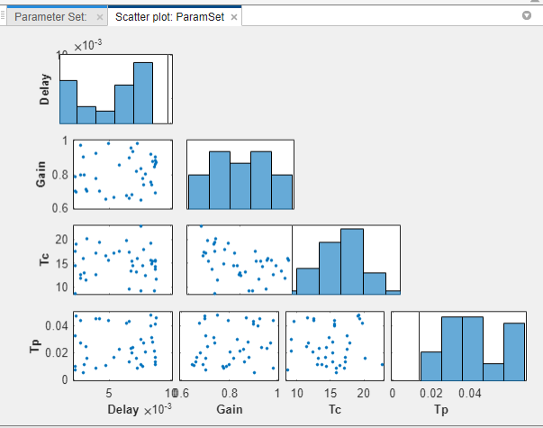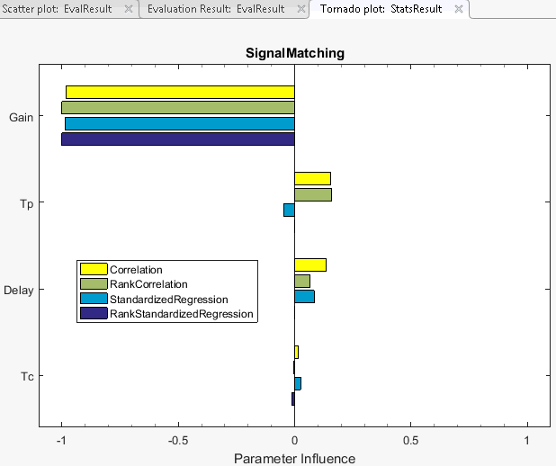## Interact with Plots in the Sensitivity Analysis Tool

This topic shows how to interact with and interpret plots generated in the Sensitivity Analysis tool.

### Parameter Set Plots

After you have generated parameter values for sensitivity analysis, you can plot the generated parameter set. For information about parameter generation, see Generate Parameter Samples for Sensitivity Analysis.

The tool displays the generated parameter set and the corresponding parameter set table. The number of rows in the parameter set table correspond to the number of samples you specified. To plot the generated parameters in the tool:

1. Select the generated parameter set in the Parameter Sets area of the tool.2. On the Plots tab, select Scatter Plot.

Alternatively, right-click the parameter set, and select Plot in the drop-down menu.

The diagonal subplots display the histograms of generated parameter values. The off-diagonal subplots are pair-wise scatter plots of the parameters. The number of data points in each scatter plot equals the number of rows in the parameter set table.You can inspect the histograms to ensure that the generated parameter values match the desired parameter distributions within the constraints of a finite sample size. Inspect the off-diagonal scatter plots to ensure that any specified correlations between parameters are present. For more information, see Inspect the Generated Parameter Set.

3. To access additional plot features, right-click in the white area of any scatter plot.

You can choose from the following options:

• Variables — Select the parameters to plot.

• Groups — Select grouping variables for the plots, and configure how the groups are displayed.

To select a parameter as a grouping variable, click Groups > New Grouping Variable. For example, the following plot is generated when the grouping variable is `Gain`.The tool creates three groups based on low, medium, and high values of the grouping variable. The tool computes these grouping values, but you can change them in the Manage Groups dialog box. The second diagonal plot shows the distribution of the gain values in the low (blue), medium (red), and high (yellow) groups. The other diagonal plots show the distribution of the remaining parameters when the corresponding gain value is low, medium, or high. The off-diagonal scatter plots show points belonging to the same group using the same color.

You can demarcate the groups based on marker size and marker type instead of color, add more groups corresponding to the grouping variable, and change the grouping values. You can also add more grouping variables. To do so, click Groups > Manage Groups.In the Manage Groups dialog box, you configure how the groups are displayed. You can perform tasks such as:

• Select the plotting Style as either `Color`, `MarkerSize`, or `MarkerType`. In the plots, the tool uses the selected style to demarcate the groups corresponding to a grouping variable.

• Select whether a grouping variable is Active. If a grouping variable is inactive, the scatter plot points are not demarcated in groups corresponding to that variable. To delete a grouping variable, clickin the corresponding Remove column.

• Add more grouping variables using the Create Grouping Variable drop-down list.

• For a grouping variable, specify the range of values for each group in the Bin/Value column. For example, currently the dialog box shows that the `Gain` values in the groups are:

• Low — below 0.7736

• Medium — 0.7736–0.8265

• High — above 0.8265

To change the Low group values to be 0.79 or lower, type `0.79` in the corresponding row of the Bin/Value column.

• Add more groups corresponding to a grouping variable. For example, to add a group with values from 0.8265 through 0.9, type `0.9` in New Bin/Value, and click .

• Upper triangle plot — Plot the off-diagonal subplots above the diagonal in addition to the existing plots.

• Marginal Box Plots — Requires Statistics and Machine Learning Toolbox™ software. Plot box plots for each of the parameters in the parameter set, and choose the position of the plots.

• Histograms — Plot the probability distribution of the parameters, and choose the position of the plots.

• Kernel Density Plots — Requires Statistics and Machine Learning Toolbox software. Plot the probability distribution of the parameters using a kernel density estimator, and choose the position of the plots. For more information, see Kernel Distribution (Statistics and Machine Learning Toolbox).

• Overlay linear fit — Plot the best-fit line on the scatter subplots. You can choose to plot the best-fit lines for one, all, a row, or a column of scatter subplots.

• Enable brushing/data selection — Enable selection of data points in the scatter subplots.When you highlight parameter values in one plot, the values corresponding to other parameters from the same row in the parameter set table are also highlighted. In addition, the rows in the parameter set table that correspond to these values are highlighted.To remove the highlighting, invert the selection to all other data points in the plot, or disable the feature, right-click the highlighted data points, and choose from the context-menu.

• Pop-out plot — View a subplot in a new window.

### Requirement Plots

After you have specified design requirements, you can plot the requirements and associated model response. For information about specifying the requirements, see Specify Time-Domain Requirements and Specify Frequency-Domain Requirements.

The specified requirements are displayed in the Requirements area of the tool. To plot the requirement in the tool, right-click the requirement, and select Plot.Alternatively, select the requirement, and in the Plots tab of the tool, select the plot type. A plot is generated and a new tab associated with the plot appears in the tool. In the new tab, you can perform additional tasks such as preprocessing imported data (for signal matching requirement only), zooming, and plotting the associated model response. The model response is the signal or system on which the requirement is applied. The response is plotted using the parameter values specified in the model workspace and is not updated during evaluation.### Evaluated Result Scatter Plots

After you have evaluated your design requirements, an evaluation results table lists the samples in the parameter set and the corresponding evaluated requirement (cost function) values. For requirements that involve a bound, a positive requirement value indicates that your requirement was violated, while a negative value indicates that the requirement was satisfied for that sample of parameter values.An evaluation result plot is also generated. The scatter subplots display the evaluated requirement (cost function value) as a function of each parameter in the parameter set. The number of points in each scatter plot equals the number of rows in the parameter set. The last column of subplots displays histograms of the probability distribution of the evaluated cost function values.Use this plot to visually analyze the relation between parameters and requirements. For example, in this case, the `SignalMatching` requirement looks monotonically related to the `Gain` parameter.

You can also plot best-fit lines on the scatter subplots. To do so, and to access additional plot features, right-click in the white area of any scatter subplot.

You can choose from the following options:

• X-Variables — Select the parameters and requirements to use as x-variables in the scatter subplots.

• Y-Variables — Select the parameters and requirements to use as y-variables in the scatter subplots.

• Grouping — Select grouping variables for the subplots, and configure how the groups are displayed.

To select a parameter or evaluated requirement as a grouping variable, click Groups > New Grouping Variable. For example, the following plot is generated when the grouping variable is `Gain`. The tool creates three groups based on low, medium, and high values of the grouping variable. The tool computes these grouping values, but you can change them in the Manage Groups dialog box. The scatter subplots display the evaluated requirement values when the corresponding gain value is low (blue), medium (red), and high (yellow). The histogram plots the probability distribution of the evaluated requirement corresponding to the groups.You can demarcate the groups based on marker size and marker type instead of color, add more groups corresponding to the grouping variable, and change the grouping values. You can also add more grouping variables. To do so, click Groups > Manage Groups. For more information, see Parameter Set Plots.

• Marginal Box Plots — Requires Statistics and Machine Learning Toolbox software. Plot box plots for each of the parameters in the parameter set, and choose the position of the plots.

• Histograms — Plot the probability distribution of the parameters, and choose the position of the plots.

• Kernel Density Plots — Requires Statistics and Machine Learning Toolbox software. Plot the probability distribution of the parameters using a kernel density estimator, and choose the position of the plots. For more information, see Kernel Distribution (Statistics and Machine Learning Toolbox).

• Overlay linear fit — Plot the best-fit line on the scatter subplots. You can choose to plot the best-fit lines for one, all, a row, or a column of scatter plots.

• Enable brushing/data selection — Enable selection of data points in the scatter subplots.When you highlight parameter values in one scatter subplot, the values corresponding to other parameters from the same row in the evaluated results table are also highlighted. In addition, the rows in the evaluated results table that correspond to these values are highlighted.To remove the highlighting, invert the selection to all other data points in the plot, or disable the feature, right-click the highlighted data points, and choose from the context-menu.

• Pop-out plot — View a subplot in a new window.

### Evaluated Result Contour Plots

After you have evaluated your design requirements, an evaluation results table lists the samples in the parameter set and the corresponding evaluated requirement (cost function) values. For information about evaluation, see Evaluate Design Requirements.

You can plot a contour plot of the evaluated results. To do so, select the evaluated result in the Results area of the tool, and choose a contour plot in the Plots tab of the tool.Use this plot to visually analyze the relation between parameters and design requirements. Select the parameters to plot in the X parameter and Y parameter drop-down lists. The evaluated requirement value is plotted as a function of these parameters.

After you have evaluated the design requirements for each parameter, you can perform statistical analysis to analyze how the parameters of your Simulink® model influence the requirements.

To generate a tornado plot ranking the influence of parameters on requirements:

1. In the Statistics tab of the tool, select the evaluation results you want to analyze in the Evaluation Results to Analyze list.2. Specify the statistical analysis methods.

You can choose to calculate a correlation coefficient, standardized regression coefficient, and partial correlation coefficient (requires Statistics and Machine Learning Toolbox software).

3. For each of these methods, specify what data to use for the analysis. You can choose from Linear (Pearson), Ranked (Spearman), and Kendall analysis types. Kendall is applicable when the analysis method is Correlation, and requires Statistics and Machine Learning Toolbox software.

You can compute all applicable combinations of analysis methods and types.

4. Calculate the coefficients, and generate a tornado plot.

Click .

The resulting tornado plot displays the calculated coefficients for each specified analysis method and type. The coefficients are plotted in order of influence of parameters on the cost function. The parameter with the greatest magnitude of influence on the cost function is displayed on the top, giving the plot a tornado shape. When more than one type of coefficient is calculated, the tornado plot sorts the parameters based on the first calculated coefficient. The coefficients are calculated in the following order:

• Correlation

• Rank correlation

• Kendall correlation

• Partial correlation

• Rank partial correlation

• Standardized regression

• Rank Standardized Regression

In this tornado plot, the parameters are sorted based on the Correlation coefficient. For all calculated coefficients, the `Gain` parameter has the most influence on the design requirement cost function.## Related Topics